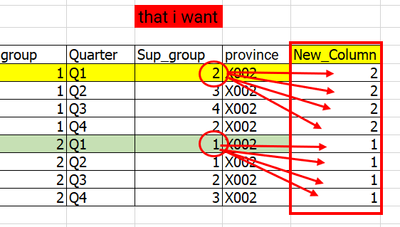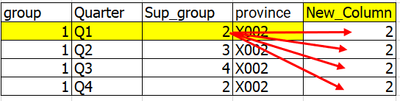cancel
Showing results for
Did you mean:Frequent Visitor

## help me please!! need to create a new column based on other columns in power bi

table exampleI need thisThe DAX I wrote is

New_Column = CALCULATE(MIN('table'[Sup_group]),FILTER(ALL('table'),
'table'[province] = EARLIER('table'[province]) &&
'Stable'[Quarter] = "Q1"))

The result isBut I don't want this kind of result, I want like the first picture.

thank you.

1 ACCEPTED SOLUTIONSuper User

Hi @only_me321

``````New_Column =
MINX (
TOPN (
1,
CALCULATETABLE ( 'table', ALLEXCEPT ( 'table', 'table'[province] ) ),
'Stable'[Quarter], ASC
),
'table'[Sup_group]
)``````
3 REPLIES 3Super User

Hi @only_me321

``````New_Column =
MINX (
TOPN (
1,
CALCULATETABLE ( 'table', ALLEXCEPT ( 'table', 'table'[province] ) ),
'Stable'[Quarter], ASC
),
'table'[Sup_group]
)``````Frequent Visitor

Oh, I can do it, just like you let me try. 😍Frequent Visitor

thank you, I tried to follow you result iswhich i want like this